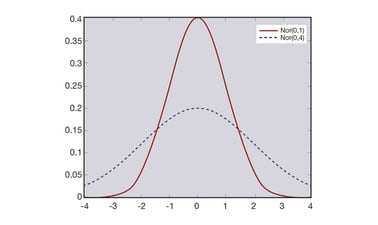# Probability and Statistics III: A Gentle Introduction to Statistics

This course provides an introduction to basic statistical concepts. We begin by walking through a library of probability distributions – including the normal distribution, which in turn leads to the Central Limit Theorem. We then discuss elementary descriptive statistics and estimation methods.

...